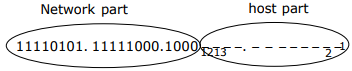# CS GATE 2012 - Online Test

Q1. Choose the grammatically INCORRECT sentence:
Explaination / Solution:
No Explaination.

Q2. In the IPv4 addressing format, the number of networks allowed under Class C addresses is
Explaination / Solution:

For class C address, size of network field is 24 bits. But first 3 bits are fixed as 110; hence total number of networks possible is 221

Q3. Which of the following transport layer protocols is used to support electronic mail?
Explaination / Solution:

E-mail uses SMTP, application layer protocol which intern uses TCP transport layer protocol.

Q4. The protocol data unit (PDU) for the application layer in the Internet stack is
Explaination / Solution:

The PDU for Datalink layer, Network layer , Transport layer and Application layer are frame, datagram, segment and message respectively.

Q5. Consider a source computer (S) transmitting a file of size 106 bits to a destination computer (D) over a network of two routers (R1 and R2) and three links (L1, L2, and L3). L1 connects S to R1; L2 connects R1 to R2; and L3 connects R2 to D. Let each link be of length 100km. Assume signals travel over each line at a speed of 108 meters per second. Assume that the link bandwidth on each link is 1Mbps. Let the file be broken down into 1000 packets each of size 1000 bits. Find the total sum of transmission and propagation delays in transmitting the file from S to D?
Explaination / Solution:
No Explaination.

Q6. Let W(n) and A(n) denote respectively, the worst case and average case running time of an algorithm executed on an input of size n. Which of the following is ALWAYS TRUE?
Explaination / Solution:

The average case time can be lesser than or even equal to the worst case. So A(n) would be upper bounded by W(n) and it will not be strict upper bound as it can even be same (e.g. Bubble Sort and merge sort). ∴A(n) = O(W(n))

Q7. Consider the following logical inferences.
I1 : If it rains then the cricket match will not be played. The cricket match was played. Inference: There was no rain.
I2 : If it rains then the cricket match will not be played. It did not rain. Inference: The cricket match was played.
Which of the following is TRUE?
Explaination / Solution:
No Explaination.

Q8. An Internet Service Provider (ISP) has the following chunk of CIDR-based IP addresses available with it: 245.248.128.0/20. The ISP wants to give half of this chunk of addresses to Organization A, and a quarter to Organization B, while retaining the remaining with itself. Which of the following is a valid allocation of address to A and B?
Explaination / Solution:Since half of 4096 host addresses must be given to organization A, we can set 12th bit to 1 and include that bit into network part of organization A, so the valid allocation of addresses to A is 245.248.136.0/21
Now for organization B, 12th bit is set to ‘0’ but since we need only half of 2048 addresses, 13th bit can be set to ‘0’ and include that bit into network part of organization B so the valid allocation of addresses to B is 245.248.128.0/22

Q9. Register renaming is done is pipelined processors
Explaination / Solution:

Register renaming is done to eliminate WAR/WAW hazards.

Q10. The recurrence relation capturing the optimal execution time of the Towers of Hanoi problem with n discs is
Explaination / Solution:

Let the three pegs be A,B and C, the goal is to move n pegs from A to C using peg B The following sequence of steps are executed recursively 1.move n−1 discs from A to B. This leaves disc n alone on peg A --- T(n-1) 2.move disc n from A to C---------1 3.move n−1 discs from B to C so they sit on disc n----- T(n-1) So, T(n) = 2T(n-1) +1